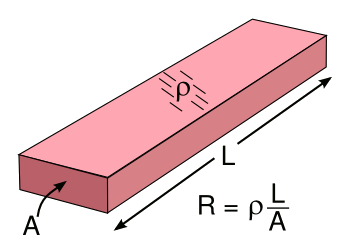### Resistor Networks

September 26, 2016

While most audio signal processing is now done using digital circuitry, it's often easier in some cases to implement simple audio filters using venerable analog circuit techniques. While using an analog bandpass filter in a recent circuit design, I ran into the common problem of needing an uncommon resistance value to hit the desired frequency.

I did what most electrical engineers do in that case, I used a parallel combination of two resistors. The problem could have been solved, also, by a series combination of two resistors. In my case, a parallel combination of a 470 k-ohm and a 1 meg-ohm resistor gave a resistance of 320 k-ohms, close enough to my desired 330 k-ohms. The formulas for calculating series and parallel resistance are found in the figure, below.Resistors in series and parallel combination.

In the series case, the resistance R is calculated as the sum of the individual resistances. In a parallel combination, the conductance, which is the reciprocal of the resistance, is summed.

(Created using Inkscape.)

It's easy to validate these formulas by looking at how resistance arises at the physical level. The resistance R of a regularly shaped piece of a material with resistivity ρ, cross-sectional area A and length L, as shown in the figure, is just R = ρ (L/A).Resistance formula for a conductor with resistivity, ρ.

(Illustration by the author using
Inkscape.)

When we put resistors in series, we're adding the lengths of their internal conductors, so the simple addition suffices. When we put resistors in parallel, we're adding the cross-sectional areas. Inverting the resistance formula allows an addition of the areas. These concepts of series and parallel resistance can take us quite far in the analysis of resistor networks, as shown in the two different connections of resistors between three terminals, as shown in the figure.Y-Delta resistor networks and their equivalent resistor values. (Created using Inkscape.)

The transformation of values in these two networks was first published by Arthur Edwin Kennelly in 1899. Kennelly was the thesis advisor of Vannevar Bush at MIT. It's easy to see how the resistance between nodes in the delta configuration (left) can be calculated as a parallel combination of a resistor and two series resistors. In the Y configuration (right), a series combination is all that's needed. Some lengthy, but not too advanced, algebra is all that's needed to find which values of resistors will give the equivalent resistance between the nodes.

Since I'm an experimentalist at heart, I decided to test these equations, first connecting nominal 1 k-ohm, 2.2 k-ohm, and 4.7 k-ohm resistors in the Δ-configuration. The measured values of these resistors, and the calculated resistors for the Y-configuration are listed below (values in ohms).

 RA = 996 R1 = 1315 RB = 2202 R2 = 595 RC = 4740 R3 = 276

I used variable resistors to create the values needed for the Y-configuration. The measured resistance values between nodes match quite well, as can be seen in the table. Any errors likely arise from my instrumentation, a hand-held digital volt-ohm-milliamp meter.

 Nodes Resistance(calc) Δ(meas) Y(meas) ErrorΔ (%) ErrorY (%) 1-2 1909.6 1909 1902 0.0 -0.4 2-3 871.0 871 873 0.0 0.2 3-1 1591.2 1540 1590 -3.2 -0.1

These preliminaries lead us to what I think is the most interesting network of resistors; that is, the infinite lattice of resistors shown in the figure. Adding a touch of infinity to a problem makes it both more interesting and often more complex.An infinite lattice of resistors in two dimensions, connected at each crossing. The resistance between some of the nodes will give an experimental value of pi (π). (Illustration by the author using Inkscape.)

A very nice analysis of this infinite array of resistors was published in 1999, and there have been many papers on this lattice array, similar lattice arrays, and cubic arrays, published on arXiv.[2-11] The following table shows the calculated resistance values, in units of the identical resistance values in the array, between the origin (0,0) and some nearby lattice nodes. Since this is an infinite array, the origin can be anywhere.

 (i,j) R (i,j) R 0,0 0 || 2,2 (8/3π) 0,1 1/2 || 3,3 (46/15π) 1,0 1/2 || 4,4 (352/105π) 1,1 2/π || 5,5 (1126/315π)

It's interesting that the resistance between some nodes will give rational numbers, while other nodes will give values containing π, an irrational, transcendental number.

Infinite resistor networks of this sort are useful for
modeling the conductivity of assemblages of discrete conductors. As shown in the figure, the conductivity of particle assemblages can be modeled by their internal resistance, and the resistive links to nearest neighbors. While the figure shows the two-dimensional case, there can be three-dimensional arrays. Electrical percolation can be modeled this way.Modeling electrical conductivity in a rectangular array of particles.

The model has resistive links to nearest neighbors.

Fig. 1 of ref. 12, via arXiv

### References:

Linked Keywords: Audio signal processing; digital; electronic circuit; circuitry; audio filter; analog electronics; analog circuit; bandpass filter; resistance; frequency; electrical engineering; electrical engineer; parallel combination; resistor; series combination; ohm; k-ohm; meg-ohm; formula; calculation; conductance; multiplicative inverse; reciprocal; Inkscape; physics; physical; material; resistivity; cross-sectional area; length; inverting; network analysis; analysis of resistor networks; terminal; Y-Delta transform; transformation; Arthur Edwin Kennelly; doctoral advisor; thesis advisor; Vannevar Bush; Massachusetts Institute of Technology; MIT; node; algebra; experimentalist; equation; potentiometer; variable resistor; measurement; measure; errors and residuals in statistics; instrumentation; multimeter; digital volt-ohm-milliamp meter; infinity; infinite; lattice graph; square grid graph; two-dimensional space; experiment; experimental; pi; analysis; scientific literature; publish; arXiv; origin; rational number; irrational number; transcendental number; computer simulation; modeling; Voronoi diagram; nearest neighbor; three-dimensional space; electrical percolation.